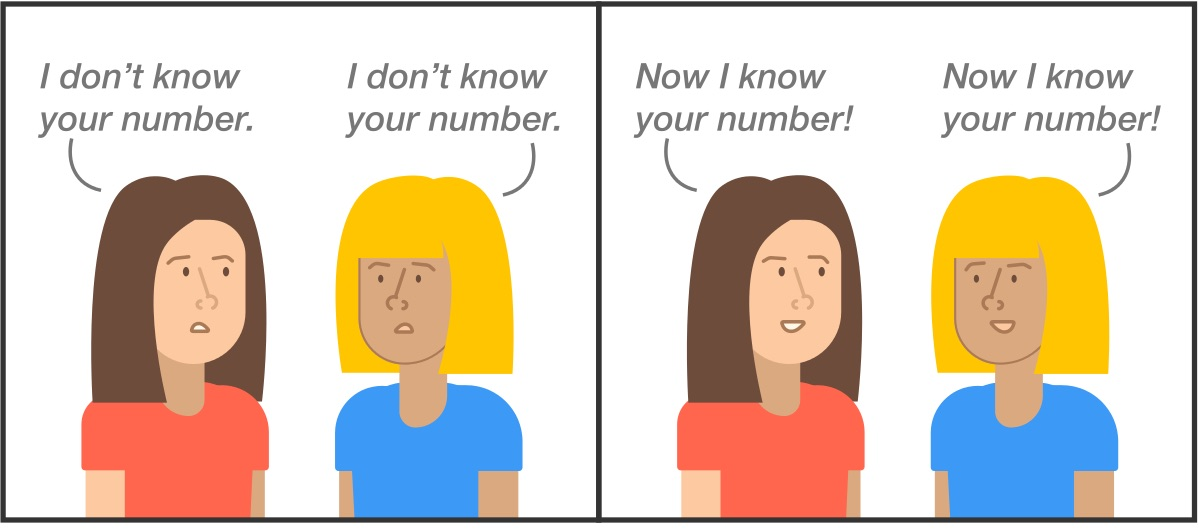# Finding "Zebedees's Number" Problem Challenging

This problem was made by: Alex Bellos This is what it said:

Zebedee secretly writes down two consecutive positive integers. Then he tells one of the numbers to Xanthe, and the other number to Yvette (without telling them each other's number, but only telling their numbers are consecutive). The following conversation ensues:Xanthe: "I don't know your number." Yvette: "I don't know your number." Xanthe: "Now I know your number!" Yvette: "Now I know your number!"

Which of the following must be one of the two numbers? 1,2,3,4,5 or it is not possible to answer. (answer was 3)

I wasn't sure wich was the correct answer. I am also not sure what a consecutive positive integer is. Could someone please explain how this problem works. Thanks!Note by Rocky E
3 years, 11 months ago

This discussion board is a place to discuss our Daily Challenges and the math and science related to those challenges. Explanations are more than just a solution — they should explain the steps and thinking strategies that you used to obtain the solution. Comments should further the discussion of math and science.

When posting on Brilliant:

• Use the emojis to react to an explanation, whether you're congratulating a job well done , or just really confused .
• Ask specific questions about the challenge or the steps in somebody's explanation. Well-posed questions can add a lot to the discussion, but posting "I don't understand!" doesn't help anyone.
• Try to contribute something new to the discussion, whether it is an extension, generalization or other idea related to the challenge.
• Stay on topic — we're all here to learn more about math and science, not to hear about your favorite get-rich-quick scheme or current world events.

MarkdownAppears as
*italics* or _italics_ italics
**bold** or __bold__ bold
- bulleted- list
• bulleted
• list
1. numbered2. list
1. numbered
2. list
Note: you must add a full line of space before and after lists for them to show up correctly
paragraph 1paragraph 2

paragraph 1

paragraph 2

[example link](https://brilliant.org)example link
> This is a quote
This is a quote
    # I indented these lines
# 4 spaces, and now they show
# up as a code block.

print "hello world"
# I indented these lines
# 4 spaces, and now they show
# up as a code block.

print "hello world"
MathAppears as
Remember to wrap math in $$ ... $$ or $ ... $ to ensure proper formatting.
2 \times 3 $2 \times 3$
2^{34} $2^{34}$
a_{i-1} $a_{i-1}$
\frac{2}{3} $\frac{2}{3}$
\sqrt{2} $\sqrt{2}$
\sum_{i=1}^3 $\sum_{i=1}^3$
\sin \theta $\sin \theta$
\boxed{123} $\boxed{123}$

## Comments

Sort by:

Top Newest

Let the two numbers be $x$ and $y$, Xanthe's numbers is $x$ and Yvette's number is $y$. Since $x$ and $y$ are consecutive positive integers, $x+1=y$ or $y+1=x$. If $x$ is one, then $y$ only an be 2, since they are positive integers, but Xanthe didn't know first Yvette's number, so $x\neq1$. If $y=1$, then $x=2$, and if $y=2$, then $x=3$, but Yvette didn't know first Xanthne's number, so $y\neq1, 2$. Next time Xanthne knew the number, $x=3$, because then $y=4$ for sure, and if $x>3$, then there are two cases, so Xanthne wouldn't know it. So $x=3$, and $y=4$.

- 3 years, 11 months ago

Log in to reply

I think you're referring to this question.

If "x" and "x+1" are positive integers, then they are consecutive positive integers as well.

You can look at the solution discussion to see how it's done.

- 3 years, 11 months ago

Log in to reply

Thank you Áron Bán-Szabó that makes way more sense now.

- 3 years, 11 months ago

Log in to reply

Thank you also Pi Han Goh for clarifying.

- 3 years, 11 months ago

Log in to reply

×

Problem Loading...

Note Loading...

Set Loading...